# Data Visualization using Matplotlib

Data Visualization is the process of presenting data in the form of graphs or charts. It helps to understand large and complex amounts of data very easily. It allows the decision-makers to make decisions very efficiently and also allows them in identifying new trends and patterns very easily. It is also used in high-level data analysis for Machine Learning and Exploratory Data Analysis (EDA).  Data visualization can be done with various tools like Tableau, Power BI, Python.

In this article, we will discuss how to visualize data with the help of the Matplotlib library of Python.

## Matplotlib

Matplotlib is a low-level library of Python which is used for data visualization. It is easy to use and emulates MATLAB like graphs and visualization. This library is built on the top of NumPy arrays and consist of several plots like line chart, bar chart, histogram, etc. It provides a lot of flexibility but at the cost of writing more code.

## Installation

We will use the pip command to install this module. If you do not have pip installed then refer to the article, Download and install pip Latest Version.

To install Matplotlib type the below command in the terminal.

`pip install matplotlib`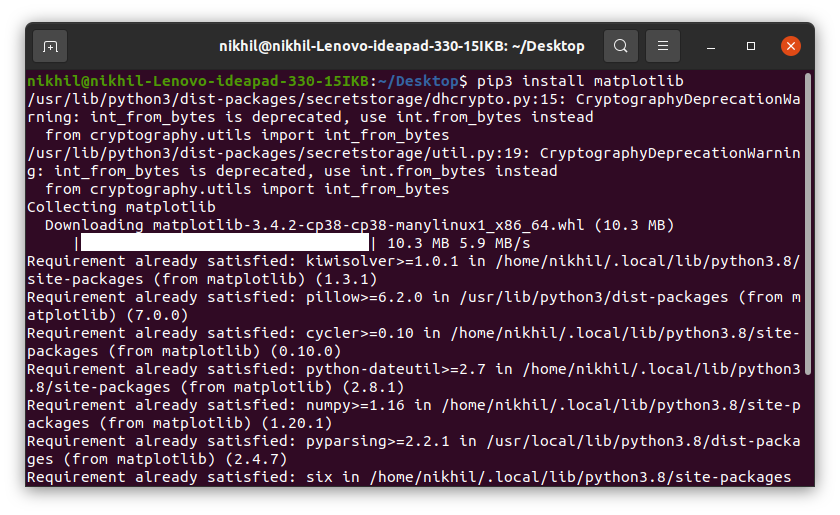Refer to the below articles to get more information setting up an environment with Matplotlib.

## Pyplot

Pyplot is a Matplotlib module that provides a MATLAB-like interface. Matplotlib is designed to be as usable as MATLAB, with the ability to use Python and the advantage of being free and open-source. Each pyplot function makes some change to a figure: e.g., creates a figure, creates a plotting area in a figure, plots some lines in a plotting area, decorates the plot with labels, etc. The various plots we can utilize using Pyplot are Line Plot, Histogram, Scatter, 3D Plot, Image, Contour, and Polar.

After knowing a brief about Matplotlib and pyplot let’s see how to create a simple plot.

Example:

## Python3

 `import` `matplotlib.pyplot as plt`   `# initializing the data` `x ``=` `[``10``, ``20``, ``30``, ``40``]` `y ``=` `[``20``, ``25``, ``35``, ``55``]`   `# plotting the data` `plt.plot(x, y)`   `plt.show()`

Output: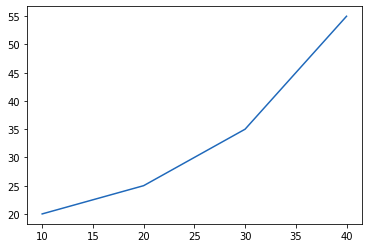Now let see how to add some basic elements like title, legends, labels to the graph.

The title() method in matplotlib module is used to specify the title of the visualization depicted and displays the title using various attributes.

Syntax:

Example:

## Python3

 `import` `matplotlib.pyplot as plt`   `# initializing the data` `x ``=` `[``10``, ``20``, ``30``, ``40``]` `y ``=` `[``20``, ``25``, ``35``, ``55``]`   `# plotting the data` `plt.plot(x, y)`   `# Adding title to the plot` `plt.title(``"Linear graph"``)`   `plt.show()`

Output: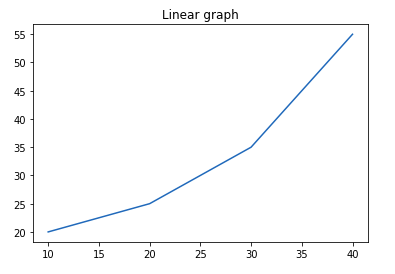We can also change the appearance of the title by using the parameters of this function.

Example:

## Python3

 `import` `matplotlib.pyplot as plt`   `# initializing the data` `x ``=` `[``10``, ``20``, ``30``, ``40``]` `y ``=` `[``20``, ``25``, ``35``, ``55``]`   `# plotting the data` `plt.plot(x, y)`   `# Adding title to the plot` `plt.title(``"Linear graph"``, fontsize``=``25``, color``=``"green"``)`   `plt.show()`

Output: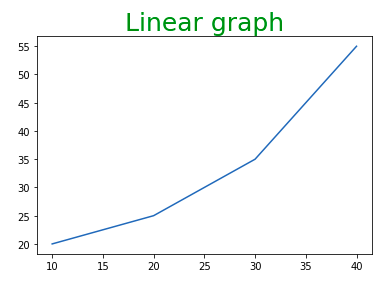## Adding X Label and Y Label

In layman’s terms, the X label and the Y label are the titles given to X-axis and Y-axis respectively. These can be added to the graph by using the xlabel() and ylabel() methods.

Syntax:

Example:

## Python3

 `import` `matplotlib.pyplot as plt`     `# initializing the data` `x ``=` `[``10``, ``20``, ``30``, ``40``]` `y ``=` `[``20``, ``25``, ``35``, ``55``]`   `# plotting the data` `plt.plot(x, y)`   `# Adding title to the plot` `plt.title(``"Linear graph"``, fontsize``=``25``, color``=``"green"``)`   `# Adding label on the y-axis` `plt.ylabel(``'Y-Axis'``)`   `# Adding label on the x-axis` `plt.xlabel(``'X-Axis'``)`   `plt.show()`

Output: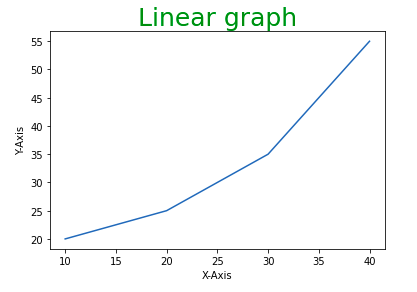## Setting Limits and Tick labels

You might have seen that Matplotlib automatically sets the values and the markers(points) of the X and Y axis, however, it is possible to set the limit and markers manually. xlim() and ylim() functions are used to set the limits of the X-axis and Y-axis respectively. Similarly, xticks() and yticks() functions are used to set tick labels.

Example: In this example, we will be changing the limit of Y-axis and will be setting the labels for X-axis.

## Python3

 `import` `matplotlib.pyplot as plt`     `# initializing the data` `x ``=` `[``10``, ``20``, ``30``, ``40``]` `y ``=` `[``20``, ``25``, ``35``, ``55``]`   `# plotting the data` `plt.plot(x, y)`   `# Adding title to the plot` `plt.title(``"Linear graph"``, fontsize``=``25``, color``=``"green"``)`   `# Adding label on the y-axis` `plt.ylabel(``'Y-Axis'``)`   `# Adding label on the x-axis` `plt.xlabel(``'X-Axis'``)`   `# Setting the limit of y-axis` `plt.ylim(``0``, ``80``) `   `# setting the labels of x-axis` `plt.xticks(x, labels``=``[``"one"``, ``"two"``, ``"three"``, ``"four"``])`   `plt.show()`

Output: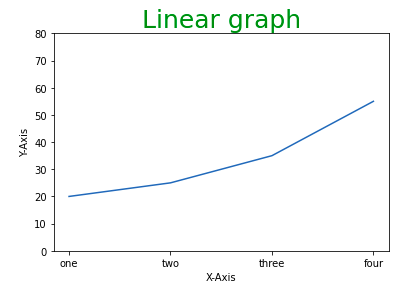A legend is an area describing the elements of the graph. In simple terms, it reflects the data displayed in the graph’s Y-axis. It generally appears as the box containing a small sample of each color on the graph and a small description of what this data means.

The attribute bbox_to_anchor=(x, y) of legend() function is used to specify the coordinates of the legend, and the attribute ncol represents the number of columns that the legend has. Its default value is 1.

Syntax:

matplotlib.pyplot.legend([“name1”, “name2”], bbox_to_anchor=(x, y), ncol=1)

Example:

## Python3

 `import` `matplotlib.pyplot as plt`     `# initializing the data` `x ``=` `[``10``, ``20``, ``30``, ``40``]` `y ``=` `[``20``, ``25``, ``35``, ``55``]`   `# plotting the data` `plt.plot(x, y)`   `# Adding title to the plot` `plt.title(``"Linear graph"``, fontsize``=``25``, color``=``"green"``)`   `# Adding label on the y-axis` `plt.ylabel(``'Y-Axis'``)`   `# Adding label on the x-axis` `plt.xlabel(``'X-Axis'``)`   `# Setting the limit of y-axis` `plt.ylim(``0``, ``80``) `   `# setting the labels of x-axis` `plt.xticks(x, labels``=``[``"one"``, ``"two"``, ``"three"``, ``"four"``])`   `# Adding legends` `plt.legend([``"GFG"``])`   `plt.show()`

Output: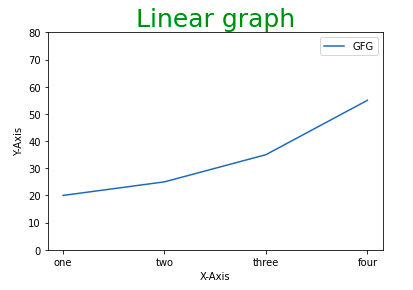Before moving any further with Matplotlib let’s discuss some important classes that will be used further in the tutorial. These classes are –

• Figure
• Axes

Note: Matplotlib take care of the creation of inbuilt defaults like Figure and Axes.

## Figure class

Consider the figure class as the overall window or page on which everything is drawn. It is a top-level container that contains one or more axes. A figure can be created using the figure() method.

Syntax:

class matplotlib.figure.Figure(figsize=None, dpi=None, facecolor=None, edgecolor=None, linewidth=0.0, frameon=None, subplotpars=None, tight_layout=None, constrained_layout=None)

Example:

## Python3

 `# Python program to show pyplot module` `import` `matplotlib.pyplot as plt` `from` `matplotlib.figure ``import` `Figure`   `# initializing the data` `x ``=` `[``10``, ``20``, ``30``, ``40``]` `y ``=` `[``20``, ``25``, ``35``, ``55``]`   `# Creating a new figure with width = 7 inches` `# and height = 5 inches with face color as ` `# green, edgecolor as red and the line width` `# of the edge as 7` `fig ``=` `plt.figure(figsize ``=``(``7``, ``5``), facecolor``=``'g'``,` `                 ``edgecolor``=``'b'``, linewidth``=``7``)`   `# Creating a new axes for the figure` `ax ``=` `fig.add_axes([``1``, ``1``, ``1``, ``1``])`   `# Adding the data to be plotted` `ax.plot(x, y)`   `# Adding title to the plot` `plt.title(``"Linear graph"``, fontsize``=``25``, color``=``"yellow"``)`   `# Adding label on the y-axis` `plt.ylabel(``'Y-Axis'``)`   `# Adding label on the x-axis` `plt.xlabel(``'X-Axis'``)`   `# Setting the limit of y-axis` `plt.ylim(``0``, ``80``) `   `# setting the labels of x-axis` `plt.xticks(x, labels``=``[``"one"``, ``"two"``, ``"three"``, ``"four"``])`   `# Adding legends` `plt.legend([``"GFG"``])`   `plt.show()`

Output: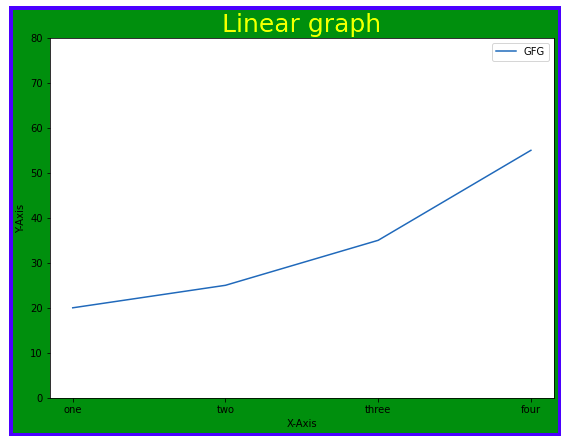>>> More Functions in Figure Class

## Axes Class

Axes class is the most basic and flexible unit for creating sub-plots. A given figure may contain many axes, but a given axes can only be present in one figure. The axes() function creates the axes object.

Syntax:

axes([left, bottom, width, height])

Just like pyplot class, axes class also provides methods for adding titles, legends, limits, labels, etc. Let’s see a few of them –

Example:

## Python3

 `# Python program to show pyplot module` `import` `matplotlib.pyplot as plt` `from` `matplotlib.figure ``import` `Figure`   `# initializing the data` `x ``=` `[``10``, ``20``, ``30``, ``40``]` `y ``=` `[``20``, ``25``, ``35``, ``55``]`   `fig ``=` `plt.figure(figsize ``=` `(``5``, ``4``))`   `# Adding the axes to the figure` `ax ``=` `fig.add_axes([``1``, ``1``, ``1``, ``1``])`   `# plotting 1st dataset to the figure` `ax1 ``=` `ax.plot(x, y)`   `# plotting 2nd dataset to the figure` `ax2 ``=` `ax.plot(y, x)`   `# Setting Title` `ax.set_title(``"Linear Graph"``)`   `# Setting Label` `ax.set_xlabel(``"X-Axis"``)` `ax.set_ylabel(``"Y-Axis"``)`   `# Adding Legend` `ax.legend(labels ``=` `(``'line 1'``, ``'line 2'``))`   `plt.show()`

Output: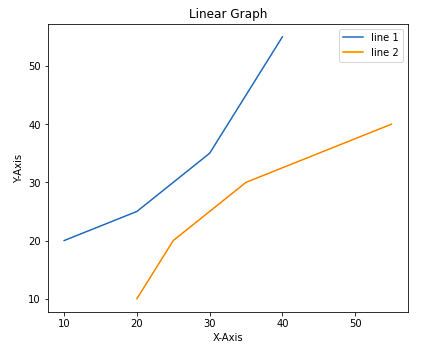## Multiple Plots

We have learned about the basic components of a graph that can be added so that it can convey more information. One method can be by calling the plot function again and again with a different set of values as shown in the above example. Now let’s see how to plot multiple graphs using some functions and also how to plot subplots.

Method 1: Using the add_axes() method

The add_axes() method is used to add axes to the figure. This is a method of figure class

Syntax:

Example:

## Python3

 `# Python program to show pyplot module` `import` `matplotlib.pyplot as plt` `from` `matplotlib.figure ``import` `Figure`   `# initializing the data` `x ``=` `[``10``, ``20``, ``30``, ``40``]` `y ``=` `[``20``, ``25``, ``35``, ``55``]`   `# Creating a new figure with width = 5 inches` `# and height = 4 inches` `fig ``=` `plt.figure(figsize ``=``(``5``, ``4``))`   `# Creating first axes for the figure` `ax1 ``=` `fig.add_axes([``0.1``, ``0.1``, ``0.8``, ``0.8``])`   `# Creating second axes for the figure` `ax2 ``=` `fig.add_axes([``1``, ``0.1``, ``0.8``, ``0.8``])`   `# Adding the data to be plotted` `ax1.plot(x, y)` `ax2.plot(y, x)`   `plt.show()`

Output: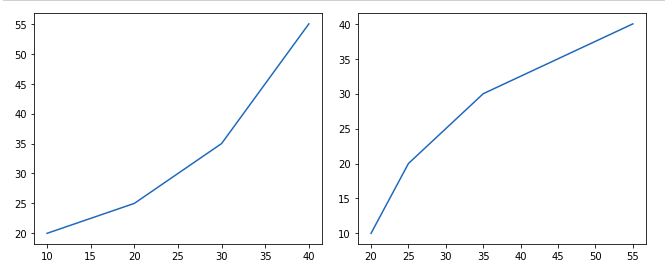Method 2: Using subplot() method.

This method adds another plot at the specified grid position in the current figure.

Syntax:

subplot(nrows, ncols, index, **kwargs)

subplot(pos, **kwargs)

subplot(ax)

Example:

## Python3

 `import` `matplotlib.pyplot as plt`     `# initializing the data` `x ``=` `[``10``, ``20``, ``30``, ``40``]` `y ``=` `[``20``, ``25``, ``35``, ``55``]`     `# Creating figure object` `plt.figure()`   `# adding first subplot` `plt.subplot(``121``)` `plt.plot(x, y)`   `# adding second subplot` `plt.subplot(``122``)` `plt.plot(y, x)`

Output: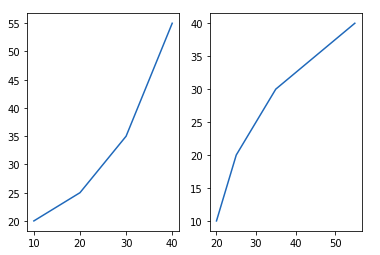Method 3: Using subplots() method

This function is used to create figures and multiple subplots at the same time.

Syntax:

matplotlib.pyplot.subplots(nrows=1, ncols=1, sharex=False, sharey=False, squeeze=True, subplot_kw=None, gridspec_kw=None, **fig_kw)

Example:

## Python3

 `import` `matplotlib.pyplot as plt`     `# initializing the data` `x ``=` `[``10``, ``20``, ``30``, ``40``]` `y ``=` `[``20``, ``25``, ``35``, ``55``]`   `# Creating the figure and subplots` `# according the argument passed` `fig, axes ``=` `plt.subplots(``1``, ``2``)`   `# plotting the data in the` `# 1st subplot` `axes[``0``].plot(x, y)`   `# plotting the data in the 1st` `# subplot only` `axes[``0``].plot(y, x)`   `# plotting the data in the 2nd ` `# subplot only` `axes[``1``].plot(x, y)`

Output: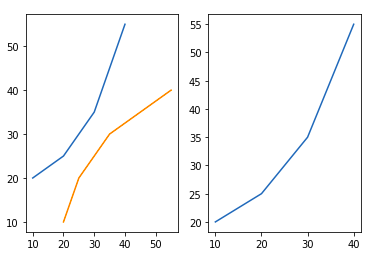Method 4: Using subplot2grid() method

This function creates axes object at a specified location inside a grid and also helps in spanning the axes object across multiple rows or columns. In simpler words, this function is used to create multiple charts within the same figure.

Syntax:

Plt.subplot2grid(shape, location, rowspan, colspan)

Example:

## Python3

 `import` `matplotlib.pyplot as plt`   `# initializing the data` `x ``=` `[``10``, ``20``, ``30``, ``40``]` `y ``=` `[``20``, ``25``, ``35``, ``55``]`   `# adding the subplots` `axes1 ``=` `plt.subplot2grid (` `(``7``, ``1``), (``0``, ``0``), rowspan ``=` `2``, colspan ``=` `1``)`   `axes2 ``=` `plt.subplot2grid (` `(``7``, ``1``), (``2``, ``0``), rowspan ``=` `2``, colspan ``=` `1``)`   `# plotting the data` `axes1.plot(x, y)` `axes2.plot(y, x)`

Output: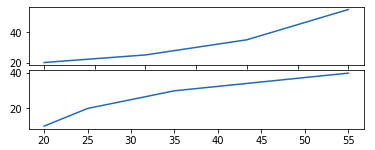## Different types of Matplotlib Plots

Matplotlib supports a variety of plots including line charts, bar charts, histograms, scatter plots, etc. We will discuss the most commonly used charts in this article with the help of some good examples and will also see how to customize each plot.

Note: Some elements like axis, color are common to each plot whereas some elements are pot specific.

### Line Chart

Line chart is one of the basic plots and can be created using the plot() function. It is used to represent a relationship between two data X and Y on a different axis.

Syntax:

matplotlib.pyplot.plot(\*args, scalex=True, scaley=True, data=None, \*\*kwargs)

Example:

## Python3

 `import` `matplotlib.pyplot as plt`     `# initializing the data` `x ``=` `[``10``, ``20``, ``30``, ``40``]` `y ``=` `[``20``, ``25``, ``35``, ``55``]`   `# plotting the data` `plt.plot(x, y)`   `# Adding title to the plot` `plt.title(``"Line Chart"``)`   `# Adding label on the y-axis` `plt.ylabel(``'Y-Axis'``)`   `# Adding label on the x-axis` `plt.xlabel(``'X-Axis'``)`   `plt.show()`

Output: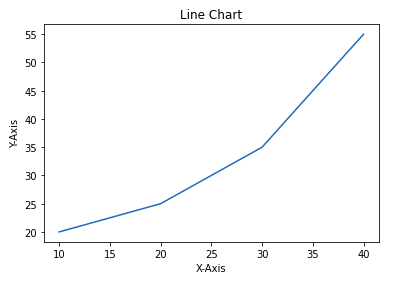Let’s see how to customize the above-created line chart. We will be using the following properties –

• color: Changing the color of the line
• linewidth: Customizing the width of the line
• marker: For changing the style of actual plotted point
• markersize: For changing the size of the markers
• linestyle: For defining the style of the plotted line

Different Linestyle available

Example:

## Python3

 `import` `matplotlib.pyplot as plt`     `# initializing the data` `x ``=` `[``10``, ``20``, ``30``, ``40``]` `y ``=` `[``20``, ``25``, ``35``, ``55``]`   `# plotting the data` `plt.plot(x, y, color``=``'green'``, linewidth``=``3``, marker``=``'o'``, ` `         ``markersize``=``15``, linestyle``=``'--'``)`   `# Adding title to the plot` `plt.title(``"Line Chart"``)`   `# Adding label on the y-axis` `plt.ylabel(``'Y-Axis'``)`   `# Adding label on the x-axis` `plt.xlabel(``'X-Axis'``)`   `plt.show()`

Output: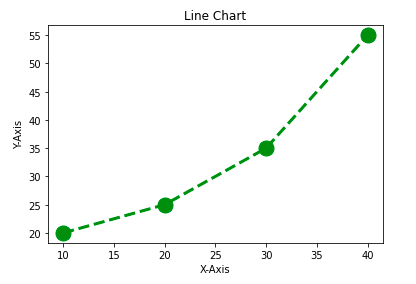### Bar Chart

A bar chart is a graph that represents the category of data with rectangular bars with lengths and heights that is proportional to the values which they represent. The bar plots can be plotted horizontally or vertically. A bar chart describes the comparisons between the discrete categories. It can be created using the bar() method.

In the below example, we will use the tips dataset. Tips database is the record of the tip given by the customers in a restaurant for two and a half months in the early 1990s. It contains 6 columns as total_bill, tip, sex, smoker, day, time, size.

Example:

## Python3

 `import` `matplotlib.pyplot as plt` `import` `pandas as pd`   `# Reading the tips.csv file` `data ``=` `pd.read_csv(``'tips.csv'``)`   `# initializing the data` `x ``=` `data[``'day'``]` `y ``=` `data[``'total_bill'``]`   `# plotting the data` `plt.bar(x, y)`   `# Adding title to the plot` `plt.title(``"Tips Dataset"``)`   `# Adding label on the y-axis` `plt.ylabel(``'Total Bill'``)`   `# Adding label on the x-axis` `plt.xlabel(``'Day'``)`   `plt.show()`

Output: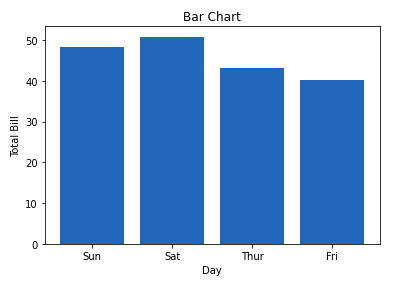Customization that is available for the Bar Chart –

• color: For the bar faces
• edgecolor: Color of edges of the bar
• linewidth: Width of the bar edges
• width: Width of the bar

Example:

## Python3

 `import` `matplotlib.pyplot as plt` `import` `pandas as pd`   `# Reading the tips.csv file` `data ``=` `pd.read_csv(``'tips.csv'``)`   `# initializing the data` `x ``=` `data[``'day'``]` `y ``=` `data[``'total_bill'``]`   `# plotting the data` `plt.bar(x, y, color``=``'green'``, edgecolor``=``'blue'``, ` `        ``linewidth``=``2``)`   `# Adding title to the plot` `plt.title(``"Tips Dataset"``)`   `# Adding label on the y-axis` `plt.ylabel(``'Total Bill'``)`   `# Adding label on the x-axis` `plt.xlabel(``'Day'``)`   `plt.show()`

Output: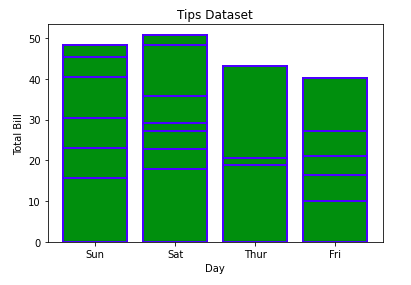Note: The lines in between the bars refer to the different values in the Y-axis of the particular value of the X-axis.

### Histogram

A histogram is basically used to represent data provided in a form of some groups. It is a type of bar plot where the X-axis represents the bin ranges while the Y-axis gives information about frequency. The hist() function is used to compute and create histogram of x.

Syntax:

matplotlib.pyplot.hist(x, bins=None, range=None, density=False, weights=None, cumulative=False, bottom=None, histtype=’bar’, align=’mid’, orientation=’vertical’, rwidth=None, log=False, color=None, label=None, stacked=False, \*, data=None, \*\*kwargs)

Example:

## Python3

 `import` `matplotlib.pyplot as plt` `import` `pandas as pd`   `# Reading the tips.csv file` `data ``=` `pd.read_csv(``'tips.csv'``)`   `# initializing the data` `x ``=` `data[``'total_bill'``]`   `# plotting the data` `plt.hist(x)`   `# Adding title to the plot` `plt.title(``"Tips Dataset"``)`   `# Adding label on the y-axis` `plt.ylabel(``'Frequency'``)`   `# Adding label on the x-axis` `plt.xlabel(``'Total Bill'``)`   `plt.show()`

Output: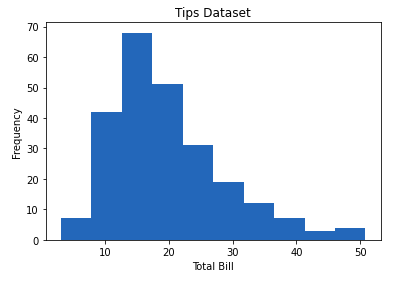Customization that is available for the Histogram –

• bins: Number of equal-width bins
• color: For changing the face color
• edgecolor: Color of the edges
• linestyle: For the edgelines
• alpha: blending value, between 0 (transparent) and 1 (opaque)

Example:

## Python3

 `import` `matplotlib.pyplot as plt` `import` `pandas as pd`   `# Reading the tips.csv file` `data ``=` `pd.read_csv(``'tips.csv'``)`   `# initializing the data` `x ``=` `data[``'total_bill'``]`   `# plotting the data` `plt.hist(x, bins``=``25``, color``=``'green'``, edgecolor``=``'blue'``,` `         ``linestyle``=``'--'``, alpha``=``0.5``)`   `# Adding title to the plot` `plt.title(``"Tips Dataset"``)`   `# Adding label on the y-axis` `plt.ylabel(``'Frequency'``)`   `# Adding label on the x-axis` `plt.xlabel(``'Total Bill'``)`   `plt.show()`

Output: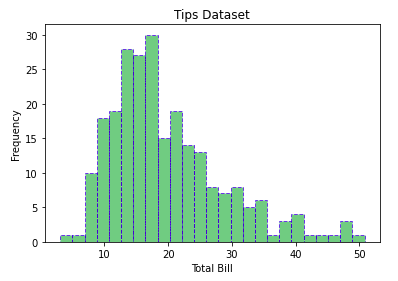### Scatter Plot

Scatter plots are used to observe relationships between variables. The scatter() method in the matplotlib library is used to draw a scatter plot.

Syntax:

matplotlib.pyplot.scatter(x_axis_data, y_axis_data, s=None, c=None, marker=None, cmap=None, vmin=None, vmax=None, alpha=None, linewidths=None, edgecolors=None

Example:

## Python3

 `import` `matplotlib.pyplot as plt` `import` `pandas as pd`   `# Reading the tips.csv file` `data ``=` `pd.read_csv(``'tips.csv'``)`   `# initializing the data` `x ``=` `data[``'day'``]` `y ``=` `data[``'total_bill'``]`   `# plotting the data` `plt.scatter(x, y)`   `# Adding title to the plot` `plt.title(``"Tips Dataset"``)`   `# Adding label on the y-axis` `plt.ylabel(``'Total Bill'``)`   `# Adding label on the x-axis` `plt.xlabel(``'Day'``)`   `plt.show()`

Output: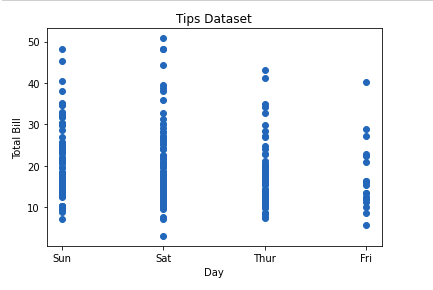Customizations that are available for the scatter plot are –

• s: marker size (can be scalar or array of size equal to size of x or y)
• c: color of sequence of colors for markers
• marker: marker style
• linewidths: width of marker border
• edgecolor: marker border color
• alpha: blending value, between 0 (transparent) and 1 (opaque)

## Python3

 `import` `matplotlib.pyplot as plt` `import` `pandas as pd`   `# Reading the tips.csv file` `data ``=` `pd.read_csv(``'tips.csv'``)`   `# initializing the data` `x ``=` `data[``'day'``]` `y ``=` `data[``'total_bill'``]`   `# plotting the data` `plt.scatter(x, y, c``=``data[``'size'``], s``=``data[``'total_bill'``],` `            ``marker``=``'D'``, alpha``=``0.5``)`   `# Adding title to the plot` `plt.title(``"Tips Dataset"``)`   `# Adding label on the y-axis` `plt.ylabel(``'Total Bill'``)`   `# Adding label on the x-axis` `plt.xlabel(``'Day'``)`   `plt.show()`

Output: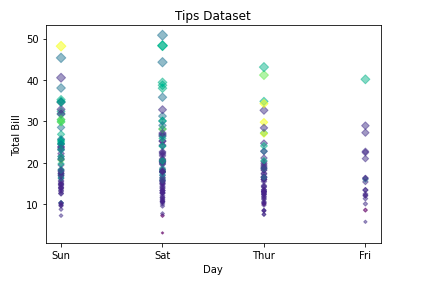### Pie Chart

Pie chart is a circular chart used to display only one series of data. The area of slices of the pie represents the percentage of the parts of the data. The slices of pie are called wedges. It can be created using the pie() method.

Syntax:

matplotlib.pyplot.pie(data, explode=None, labels=None, colors=None, autopct=None, shadow=False)

Example:

## Python3

 `import` `matplotlib.pyplot as plt` `import` `pandas as pd`   `# Reading the tips.csv file` `data ``=` `pd.read_csv(``'tips.csv'``)`   `# initializing the data` `cars ``=` `[``'AUDI'``, ``'BMW'``, ``'FORD'``,` `        ``'TESLA'``, ``'JAGUAR'``,]` `data ``=` `[``23``, ``10``, ``35``, ``15``, ``12``]`   `# plotting the data` `plt.pie(data, labels``=``cars)`   `# Adding title to the plot` `plt.title(``"Car data"``)`   `plt.show()`

Output: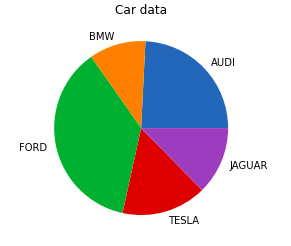Customizations that are available for the Pie chart are –

• explode: Moving the wedges of the plot
• autopct: Label the wedge with their numerical value.
• color: Attribute is used to provide color to the wedges.

Example:

## Python3

 `import` `matplotlib.pyplot as plt` `import` `pandas as pd`   `# Reading the tips.csv file` `data ``=` `pd.read_csv(``'tips.csv'``)`   `# initializing the data` `cars ``=` `[``'AUDI'``, ``'BMW'``, ``'FORD'``,` `        ``'TESLA'``, ``'JAGUAR'``,]` `data ``=` `[``23``, ``13``, ``35``, ``15``, ``12``]`   `explode ``=` `[``0.1``, ``0.5``, ``0``, ``0``, ``0``]`   `colors ``=` `( ``"orange"``, ``"cyan"``, ``"yellow"``,` `          ``"grey"``, ``"green"``,)`   `# plotting the data` `plt.pie(data, labels``=``cars, explode``=``explode, autopct``=``'%1.2f%%'``,` `        ``colors``=``colors, shadow``=``True``)`   `plt.show()`

Output: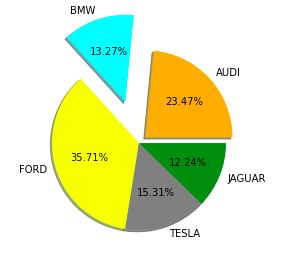## Saving a Plot

For saving a plot in a file on storage disk, savefig() method is used. A file can be saved in many formats like .png, .jpg, .pdf, etc.

Syntax:

pyplot.savefig(fname, dpi=None, facecolor=’w’, edgecolor=’w’, orientation=’portrait’, papertype=None, format=None, transparent=False, bbox_inches=None, pad_inches=0.1, frameon=None, metadata=None)

Example:

## Python3

 `import` `matplotlib.pyplot as plt`   `# Creating data` `year ``=` `[``'2010'``, ``'2002'``, ``'2004'``, ``'2006'``, ``'2008'``]` `production ``=` `[``25``, ``15``, ``35``, ``30``, ``10``]`   `# Plotting barchart` `plt.bar(year, production)`   `# Saving the figure.` `plt.savefig(``"output.jpg"``)`   `# Saving figure by changing parameter values` `plt.savefig(``"output1"``, facecolor``=``'y'``, bbox_inches``=``"tight"``,` `            ``pad_inches``=``0.3``, transparent``=``True``)`

Output: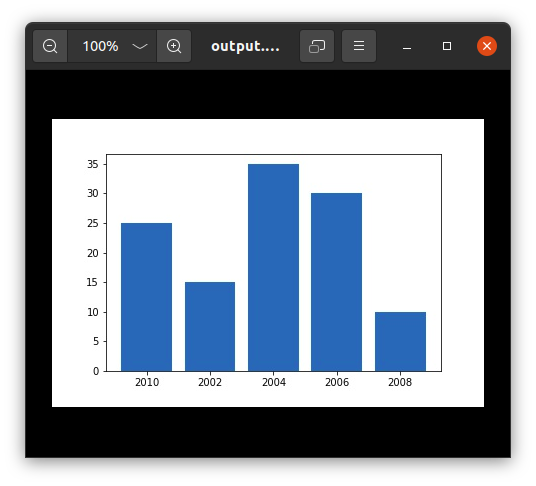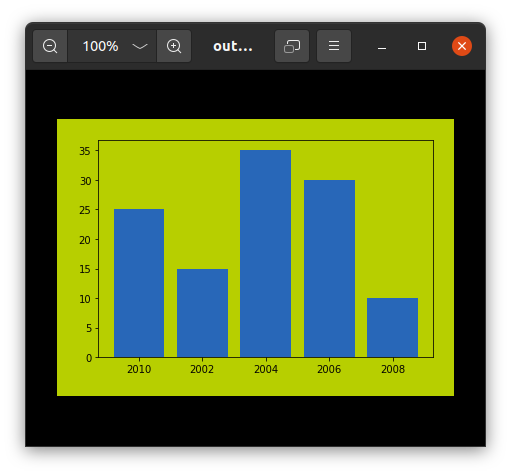Whether you're preparing for your first job interview or aiming to upskill in this ever-evolving tech landscape, GeeksforGeeks Courses are your key to success. We provide top-quality content at affordable prices, all geared towards accelerating your growth in a time-bound manner. Join the millions we've already empowered, and we're here to do the same for you. Don't miss out - check it out now!

Previous
Next